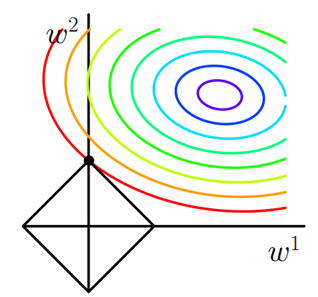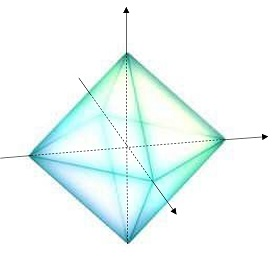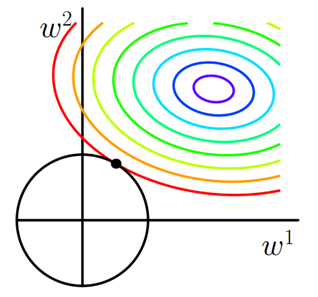# 它们的公式

l1 $l_{1}$正则项的公式为

l1=λin|θi|

l2 $l_{2}$正则项的公式为
l2=λinθ2i

J(θ)=12mi=1m(hθ(x(i))y(i))2+λj=1nθ2j

# 它们的功能

## l1 $l_1$具有“稀疏能力”

l1 $l_1$具备一个 l2 $l_2$所不具备的功能，即“稀疏能力”，即 l1 $l_1$会使参数矩阵变得稀疏，即 l1 $l_1$会倾向于使一些参数变为0，即 l1 $l_1$会倾向于减少非零参数数量。

### 凌驾于解空间之上的限制活动区

Jall=J(θ)

Jall=J(θ)+L(θ)

argminθJall=J(θ)+L(θ)

Jalllast=Jlast+Llast

argminθJall=J(θ), s.t.L(θ)Llast

### 画图理解

L(θ)=λin|θi|  Llast1. 只许在“限制活动区”活动。
2. 尽可能往“坑底”走。#### 为什么 l2 $l_2$不具备“稀疏能力”#### 除了 l1 $l_1$还有谁有稀疏能力

wi=0 $w_i=0$ 处不可导，跟“带尖儿”是一码事儿。

# 说明

08-04
08-137万+
03-0239万+
03-20384
04-052992
04-241万+
08-23576
06-01
08-03

### “相关推荐”对你有帮助么？

•非常没帮助
•没帮助
•一般
•有帮助
•非常有帮助被折叠的  条评论 为什么被折叠?到【灌水乐园】发言¥2 ¥4 ¥6 ¥10 ¥20余额支付 (余额：-- )扫码支付获取中扫码支付点击重新获取扫码支付1.余额是钱包充值的虚拟货币，按照1:1的比例进行支付金额的抵扣。
2.余额无法直接购买下载，可以购买VIP、C币套餐、付费专栏及课程。余额充值